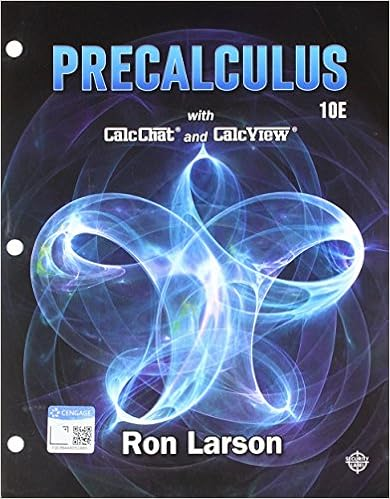Lab #1 Answers-EOQ - Module 2 Problem Set Module A certain...

• Lab Report
• 2
• 98% (249) 243 out of 249 people found this document helpful

This preview shows page 1 - 2 out of 2 pages.

We have textbook solutions for you!
The document you are viewing contains questions related to this textbook.The document you are viewing contains questions related to this textbook.
Chapter 9 / Exercise 78
Precalculus
Hostetler/LarsonExpert Verified
SCM 300 Lab #1 Answers Q1. What is the annual demand for one store? Find D: 7800 Q2. What is the per unit annual holding for one store? Find H: \$113.75 Q3. What is the per unit cost for each item? Find C: 325 Q4. What is the cost to place one order? Find S: 950 Q5. What is the weekly demand? Find D/52: 150 Q6. How many orders per year are being placed annually? Q=900: 8.67 Q7. What is the annual cost to purchase the items? Q=900: \$2,535,000 Q8. What is the annual ordering cost, AOC? (Q=900): 8,233 Q10. What is the total annual cost of inventory, TC? (Q=900): \$2,594,000 Q11. What is the EOQ?: 361 Q12. Using the calculated EOQ, how often will orders be placed? All answers are in weeks: 2.41 Q14. Using the calculated EOQ, what is the annual ordering cost (AOC)?: \$20,529
We have textbook solutions for you!
The document you are viewing contains questions related to this textbook.The document you are viewing contains questions related to this textbook.
Chapter 9 / Exercise 78
Precalculus
Hostetler/LarsonExpert Verified
•••